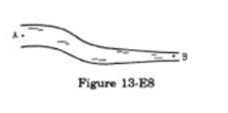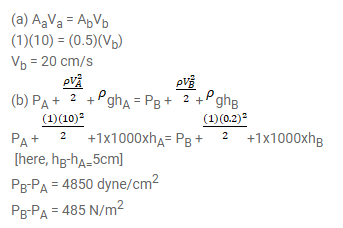# Water flows through a tube shown in figure (13-E8).

Question:

Water flows through a tube shown in figure (13-E8). The areas of cross-section at $\mathrm{A}$ and $\mathrm{B}$ are $1 \mathrm{~cm}^{2}$ and0.5 $\mathrm{cm}^{2}$ respectively. The height difference between $A$ and $B$ is $5 \mathrm{~cm}$. If the speed of water at $A$ is $10 \mathrm{~cm} / \mathrm{s}$ fid (a) the speed at $B$ (b) the difference in pressures at $\mathrm{A}$ and $\mathrm{B}$.Solution: